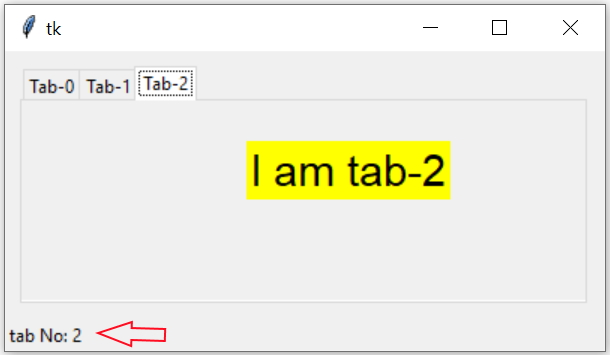# <<NotebookTabChanged>> Event on Chage of tab

Tkinter NotebookTkinter Notebook methods to select hide forget tabs and using notebook tab change event

We can display the tab number at the footer of the window. Once the tab is changed then we can bind the event to trigger a function to read the tab_id by select() method and then getting the index of the tab by using index() method.
We can execute the function by using virtual event.
``my_tabs.bind('<<NotebookTabChanged>>',my_msg)``
Here my_msg is our function and inside this we will keep our code to read and display the tab number. Note the two methods used here, `my_tabs.select()` returns the tab_id of the current tab. Then same is used as input to `my_tabs.index()` to get the tab number as integer. The function str() is used to convert the number into string before displaying at Label l4.
``````def my_msg(*args):
t_nos=str(my_tabs.index(my_tabs.select()))
l4.config(text='tab No: '+ t_nos)``````
Full code is here
``````import tkinter  as tk
from tkinter import *
from tkinter import ttk
my_w = tk.Tk()
my_w.geometry("400x200")
my_tabs = ttk.Notebook(my_w,padding=10) # declaring

tab0 = ttk.Frame(my_tabs)
tab1 = ttk.Frame(my_tabs)
tab2 = ttk.Frame(my_tabs)

my_tabs.pack(expand = 1, fill ="both")

font1=('time',22,'normal')
l1=tk.Label(tab0,text='I am tab-0',bg='yellow',font=font1)
l1.place(relx=0.4,rely=0.2) # using place
l2=tk.Label(tab1,text='I am tab-1',bg='yellow',font=font1)
l2.place(relx=0.4,rely=0.2) # using grid
l3=tk.Label(tab2,text='I am tab-2',bg='yellow',font=font1)
l3.place(relx=0.4,rely=0.2) # using place

def my_msg(*args):
t_nos=str(my_tabs.index(my_tabs.select()))
l4.config(text='tab No: '+ t_nos)

my_tabs.bind('<<NotebookTabChanged>>',my_msg)

l4=tk.Label(my_w,text='message here')
l4.pack(side=LEFT)

my_w.mainloop()  # Keep the window open``````

Subscribe to our YouTube Channel here

## Subscribe

* indicates required
Subscribe to plus2netplus2net.com

Post your comments , suggestion , error , requirements etc here